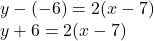need help ASAP Write an equation in whatever form you choose with the given characteristics Perpendicular to y= -1/2x – 8 and pa

Question

need help ASAP

Write an equation in whatever form you choose with the given characteristics Perpendicular to y= -1/2x – 8 and passes through: (7. -6)​

in progress 0
5 months 2021-08-25T17:09:16+00:00 1 Answers 3 views 0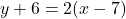2) When you know a point that the line must pass through and its slope, you can use point-slope form (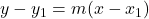) to write an equation.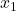and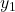represents the x and y values of the point the line intersects, and m represents the slope. So, substitute 2 for m, 7 for, and -6 for.